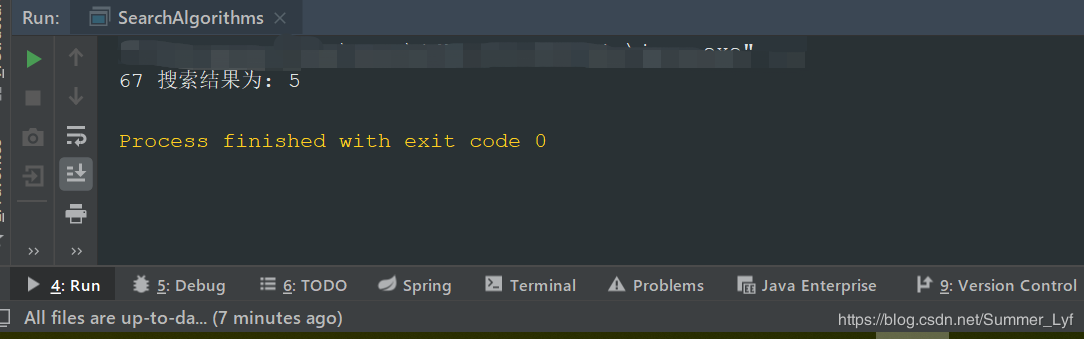欢迎访问优讯网！
 您当前的位置：首页 > 爱编程

# Java 二分法检索算法代码实现详解

 12345678910111213141516171819202122232425262728293031323334353637383940414243444546474849505152 `public` `class` `SearchAlgorithms {`` ``/**``  ``*迭代方法``  ``* @param arr``  ``* @param elementToSearch``  ``* @return``  ``*/`` ``public` `static` `int` `binarySearch(``int` `arr[], ``int` `elementToSearch) {`` ` `  ``int` `firstIndex = ``0``;``  ``int` `lastIndex = arr.length - ``1``;`` ` `  ``// 终止条件(元素不存在)``  ``while``(firstIndex <= lastIndex) {``   ``int` `middleIndex = (firstIndex + lastIndex) / ``2``;``   ``// 如果中间元素是我们的目标元素，那么返回它的索引``   ``if` `(arr[middleIndex] == elementToSearch) {``    ``return` `middleIndex;``   ``}`` ` `   ``// 如果中间的元素比较小``   ``// 将我们的指数指向中间+1，不考虑前半部分``   ``else` `if` `(arr[middleIndex] < elementToSearch)``    ``firstIndex = middleIndex + ``1``;`` ` `    ``// 如果中间的元素更大``    ``// 将我们的指数指向中间1，不考虑下半部分``   ``else` `if` `(arr[middleIndex] > elementToSearch)``    ``lastIndex = middleIndex - ``1``;`` ` `  ``}``  ``return` `-``1``;`` ``}`` ``/**``  ``* 用于打印结果``  ``* @param targetParameter``  ``* @param index``  ``*/`` ``public` `static` `void` `print(``int` `targetParameter, ``int` `index) {``  ``if` `(index == -``1``){``   ``System.out.println(targetParameter + ``" 未找到"``);``  ``}``  ``else` `{``   ``System.out.println(targetParameter + ``" 搜索结果为: "` `+ index);``  ``}`` ``}`` ``//测试一下`` ``public` `static` `void` `main(String[] args) {``  ``int` `index = binarySearch(``new` `int``[]{``89``, ``57``, ``91``, ``47``, ``95``, ``3``, ``27``, ``22``, ``67``, ``99``}, ``67``);``  ``print(``67``, index);`` ``}``}`12345678910111213141516171819202122232425262728293031323334353637383940414243444546 `public` `class` `SearchAlgorithms {`` ` ` ``/**``  ``*递归方法``  ``* @param arr``  ``* @param elementToSearch``  ``* @return``  ``*/`` ``public` `static` `int` `recursiveBinarySearch(``int` `arr[], ``int` `firstElement, ``int` `lastElement,``int` `elementToSearch) {`` ` `  ``// 结束条件``  ``if` `(lastElement >= firstElement) {``   ``int` `mid = firstElement + (lastElement - firstElement) / ``2``;`` ` `   ``// 如果中间元素是我们的目标元素，那么返回它的索引``   ``if` `(arr[mid] == elementToSearch)``    ``return` `mid;`` ` `   ``// 如果中间元素大于目标元素``   ``if` `(arr[mid] > elementToSearch)``    ``return` `recursiveBinarySearch(arr, firstElement, mid - ``1``, elementToSearch);`` ` `   ``return` `recursiveBinarySearch(arr, mid + ``1``, lastElement, elementToSearch);``  ``}`` ` `  ``return` `-``1``;`` ``}`` ``/**``  ``* 用于打印结果``  ``* @param targetParameter``  ``* @param index``  ``*/`` ``public` `static` `void` `print(``int` `targetParameter, ``int` `index) {``  ``if` `(index == -``1``){``   ``System.out.println(targetParameter + ``" 未找到"``);``  ``}``  ``else` `{``   ``System.out.println(targetParameter + ``" 搜索结果为: "` `+ index);``  ``}`` ``}`` ``//测试一下`` ``public` `static` `void` `main(String[] args) {``  ``int` `index = recursiveBinarySearch(``new` `int``[]{``3``, ``22``, ``27``, ``47``, ``57``, ``67``, ``89``, ``91``,``95``, ``99``}, ``0``, ``10``, ``67``);``  ``print(``67``, index);`` ``}``}`返回首页
 原文链接：https://www.jb51.net/article/178286.htm
 推荐资讯
 相关文章
 栏目更新
 栏目热门